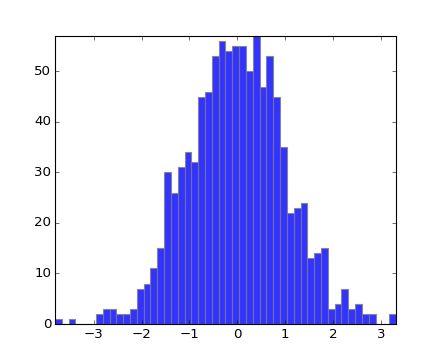### We're updating the default styles for Matplotlib 2.0

Learn what to expect in the new updates#### Previous topic

api example code: font_file.py

#### Next topic

api example code: image_zcoord.py

# api example code: histogram_path_demo.py¶```"""
This example shows how to use a path patch to draw a bunch of
rectangles.  The technique of using lots of Rectangle instances, or
the faster method of using PolyCollections, were implemented before we
had proper paths with moveto/lineto, closepoly etc in mpl.  Now that
we have them, we can draw collections of regularly shaped objects with
homogeous properties more efficiently with a PathCollection.  This
example makes a histogram -- its more work to set up the vertex arrays
at the outset, but it should be much faster for large numbers of
objects
"""

import numpy as np
import matplotlib.pyplot as plt
import matplotlib.patches as patches
import matplotlib.path as path

fig, ax = plt.subplots()

# histogram our data with numpy
data = np.random.randn(1000)
n, bins = np.histogram(data, 50)

# get the corners of the rectangles for the histogram
left = np.array(bins[:-1])
right = np.array(bins[1:])
bottom = np.zeros(len(left))
top = bottom + n

# we need a (numrects x numsides x 2) numpy array for the path helper
# function to build a compound path
XY = np.array([[left,left,right,right], [bottom,top,top,bottom]]).T

# get the Path object
barpath = path.Path.make_compound_path_from_polys(XY)

# make a patch out of it
patch = patches.PathPatch(barpath, facecolor='blue', edgecolor='gray', alpha=0.8)